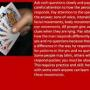# I will guess your birthday (using a math trick) #2Let me to try to explain how it works with a specific example. I am going to use the month as 8 and the day as 12 and go through the steps, but not perform all the arithmetic. 5 × 8/ 5 × 8 + 17/ 2(5 × 8 + 17) = 10 x 8 + 34/ 10 × 8 + 34 - 13 = 10 × 8 + 21/ 5(10 × 8 + 21) = 50 × 8 + 105/ 50 × 8 + 105 - 8 = 50 × 8 + 97/ 2(50 × 8 + 97) = 100 × 8 + 194/ 100 × 8 + 194 + 9 = 100 × 8 + 203/ 100 × 8 + 203 + 12/ 100 × 8 + 203 + 12 - 203 = 100 x 8 + 12/ Now see what you have. There is the number of the month (it is 8 here but it could be any month) followed by two zeros, plus the number of the day (12). So for this example I get 800 + 12 = 812.

This is the second version of (using a math trick)! I would like to thank @Jay_son for the steps and how it works, credits on him. Use a calculator for this one and the calculator will just guess your age!

Created by: Cutie_Shy

1. Multiply the number of the month in which you were born by 5
4. Subtract 13
5. Multiply by 5
6. Subtract 8